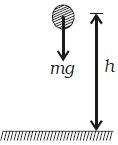# Expression for the Potential Energy

The potential energy of a body is the energy stored in the body by virtue of its position or the state of strain. Hence water stored in a reservoir, a wound spring, compressed air, stretched rubber chord, etc, possess potential energy.

Let consider a body of mass m. which is at rest at a height h above the ground as shown in Figure. The work done in raising the body from the ground to the height h is stored in the body as its potential energy and when the body falls to the ground, the same amount of work can be got back from it.Fig: Potential Energy

Now, in order to lift the body vertically up, a force mg equal to the weight of the body should be applied.

When the body is taken vertically up through a height h, then work done.

W = Force x displacement

So, W = mg x h

This work done is stored as potential energy in the body

Ep = mgh